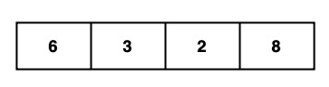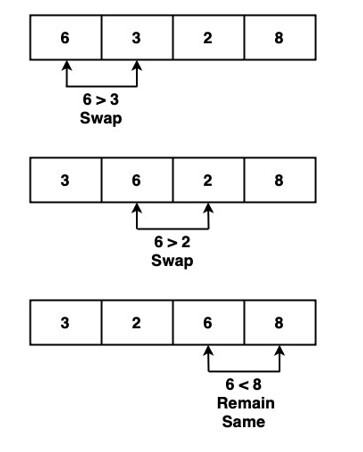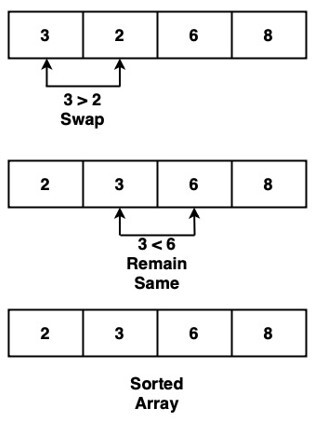# Swift Program to Implement Bubble Sort Algorithm

In swift, Bubble sort algorithm is the easiest search algorithm. This algorithm sorts the elements by repeatedly swapping adjacent elements if they are not present at the right place. This algorithm works well only for small set of elements, it is not suitable for larger number of elements because its average and worst case time complexity is high. So the working of the bubble sort is −

Suppose we have the following array −Now we sort the array in ascending order using the bubble sort. Here starting from the first index we compare the first and second element. If the first element is greater than the second element, then we swap the position of the elements with each other. Similarly compare the second and third element, if second element is greater than third element, then swap the position of the elements with each other. This process continue till the last unsorted element.

### 1st Iteration### 2nd Iteration

In the second iteration, again we have to follow the same steps till the last element.So this is how we sort the array using bubble sort.

## Algorithm

• Step 1 − Create a function to sort the array in ascending order using bubble sort algorithm.

• Step 2 − Inside the function, we run nested for-in loop to traverse over each pair of adjacent element in the given.

• Step 3 − Check if array[y]>array[y+1]. If yes, then swap the position of the elements without each other. If no, then move to next pair.

• Step 4 − Now outside the function create an array of integer type.

• Step 5 − Call the function and pass the array into it.

• Step 6 − Print the sorted array.

## Example

In the following example, we will create a function named as myBubbleSort(). This function takes an array as input and sort the given array into ascending order with the help of bubble sort algorithm. This function uses nested for-in loop to iterate through each pair of adjacent element the given array and swap if the first element is greater than the second element. This process continue till the last unsorted element. Here the function modifies the original array with the help of inout parameter. Finally display the sorted array.

import Foundation
import Glibc

// Function to sort array using bubble sort
func myBubbleSort(_ array: inout [Int]) {
let size = array.count

for x in 0..<size {
for y in 0..<size-x-1 {

if array[y] > array[y+1] {
// Swap the elements if they are
// not in the correct order
let temp = array[y]
array[y] = array[y+1]
array[y+1] = temp
}
}
}
}

// Array of integer type
var arr = [10, 87, 2, 90, 34, 1, 6, 78]
myBubbleSort(&arr)
print("Sorted array: \(arr)")


## Output

Sorted array: [1, 2, 6, 10, 34, 78, 87, 90]


## Conclusion

So this is how we can implement bubble sort algorithm. It is only suitable for small data set. The average and worst case complexity is O(n2), where n is known as the number of items.

Updated on: 21-Apr-2023

186 Views